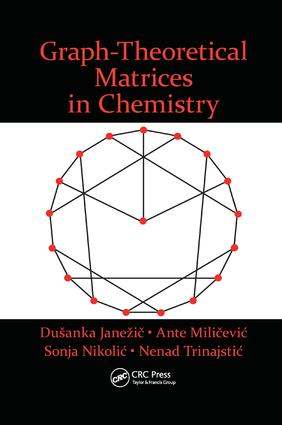# Graph-Theoretical Matrices in Chemistry

## 1st Edition

CRC Press

##### Purchasing Options:\$ = USD
Paperback: 9781138894280
pub: 2017-11-22
SAVE ~\$13.59
\$67.95
\$54.36
x
Hardback: 9781498701150
pub: 2015-04-22
SAVE ~\$35.00
\$175.00
\$140.00
x
eBook (VitalSource) : 9780429172236
pub: 2015-04-22
from \$32.48

FREE Standard Shipping!

### Description

Graph-Theoretical Matrices in Chemistry presents a systematic survey of graph-theoretical matrices and highlights their potential uses. This comprehensive volume is an updated, extended version of a former bestseller featuring a series of mathematical chemistry monographs. In this edition, nearly 200 graph-theoretical matrices are included.

This second edition is organized like the previous one—after an introduction, graph-theoretical matrices are presented in five chapters: The Adjacency Matrix and Related Matrices, Incidence Matrices, The Distance Matrix and Related Matrices, Special Matrices, and Graphical Matrices. Each of these chapters is followed by a list of references.

Among the matrices presented several are novel and some are known only to a few. The properties and potential usefulness of many of the presented graph-theoretical matrices in chemistry have yet to be investigated.

Most of the graph-theoretical matrices presented have been used as sources of molecular descriptors usually referred to as topological indices. They are particularly concerned with a special class of graphs that represents chemical structures involving molecules. Due to its multidisciplinary scope, this book will appeal to a broad audience ranging from chemistry and mathematics to pharmacology.

Introduction

References

The Adjacency Matrix and Related Matrices

The Vertex-Adjacency Matrix of Simple Graphs

The Linear Representation of the Vertex-Adjacency Matrix of Acyclic Structures

Labeling Graphs Using the Randić Procedure

The Vertex-Adjacency Matrix of Multiple Graphs

The Atom-Connectivity Matrix

The Bond-Electron Matrix

The Vertex-Adjacency Matrix of Weighted Graphs

The Vertex-Adjacency Matrix of Möbius Graphs

The Augmented Vertex-Adjacency Matrix

The Edge-Weighted Edge-Adjacency Matrix

The Burden Matrix

The Vertex-Connectivity Matrix

The Edge-Connectivity Matrix

The Sum-Vertex-Connectivity Matrix

The Sum-Edge-Connectivity Matrix

Zagreb Matrices

The Hückel Matrix

The Laplacian Matrix

The Generalized Laplacian Matrix

The Augmented Vertex-Degree Matrix

References

Incidence Matrices

The Vertex-Edge Incidence Matrix

The Edge-Vertex Incidence Matrix

The Edge-Cycle Incidence Matrix

The Cycle-Edge Incidence Matrix

The Vertex-Path Incidence Matrix

The Weighted-Hexagon-Kekulé-Structure Incidence Matrix

References

The Distance Matrix and Related Matrices

The Standard Distance Matrix or the Vertex-Distance Matrix

Generalized Vertex-Distance Matrix

The Vertex-Galvez Matrix

Combinatorial Matrices

Reciprocal Combinatorial Matrices

The Edge-Distance Matrix

The Vertex-Distance-Complement Matrix

The Augmented Vertex-Distance Matrix

The Edge-Weighted Vertex-Distance Matrix

The Barysz Vertex-Distance Matrix

The Complement of the Barysz Vertex-Distance Matrix

The Reciprocal Barysz Vertex-Distance Matrix

The Reciprocal of the Complement of the Barysz Vertex-Distance Matrix

The Complementary Vertex-Distance Matrix

The Reciprocal of the Complementary Vertex-Distance Matrix

Matrix of Dominant Distances in a Graph

The Detour Matrix

The Detour-Path Matrix

The Detour-Delta Matrix

The Edge-Weighted Detour Matrix

The Maximum-Minimum Path Matrix

The Detour-Complement Matrix

The Vertex-Distance Matrix and the Detour Matrix of Complete Graphs and Complete Bipartite Graphs

The Vertex-Harary Matrix

The Edge-Harary Matrix

The Edge-Weighted-Harary Matrix

The Modified Edge-Weighted-Harary Matrix

Distance-Degree Matrices

The Resistance-Distance Matrix

Distance/Distance Matrices

The Common Vertex Matrix

References

Special Matrices

The Distance-Sum-Connectivity Matrix

Wiener Matrices

The Modified Wiener Matrices

The Reverse-Wiener Matrix

The Reverse-Detour Matrix

Szeged Matrices

Reciprocal Szeged Matrices

The Unsymmetric Szeged Matrix

Cluj Matrices

Reciprocal Cluj Matrices

The Hosoya Matrix

The Path Matrix

The All-Path Matrix

The Expanded Vertex-Distance Matrices

The Quotient Matrices

The Random-Walk Markov Matrix

Restricted Random-Walk Matrix

The Transfer Matrix

References

Graphical Matrices

Construction of Graphical Matrices

Numerical Realization of Graphical Matrices

A Generalized Procedure for Constructing Graphical Matrices and for Obtaining Their Numerical Representations

References

Concluding Remarks

References

Index

### About the Authors

Dušanka Janežic, PhD, University of Primorska, Faculty of Mathematics, Natural Sciences and Information Technologies, Koper, Slovenia

Ante Milicevic, PhD, The Institute for Medical Research and Occupational Health, Zagreb, Croatia

Sonja Nikolic, PhD, The Rugjer Boškovic Institute, Zagreb, Croatia

Nenad Trinajstic, PhD, fellow of the Croatian Academy of Sciences and Arts, The Rugjer Boškovic Institute, Zagreb, Croatia

### Subject Categories

##### BISAC Subject Codes/Headings:
MAT036000
MATHEMATICS / Combinatorics
SCI013050
SCIENCE / Chemistry / Physical & Theoretical